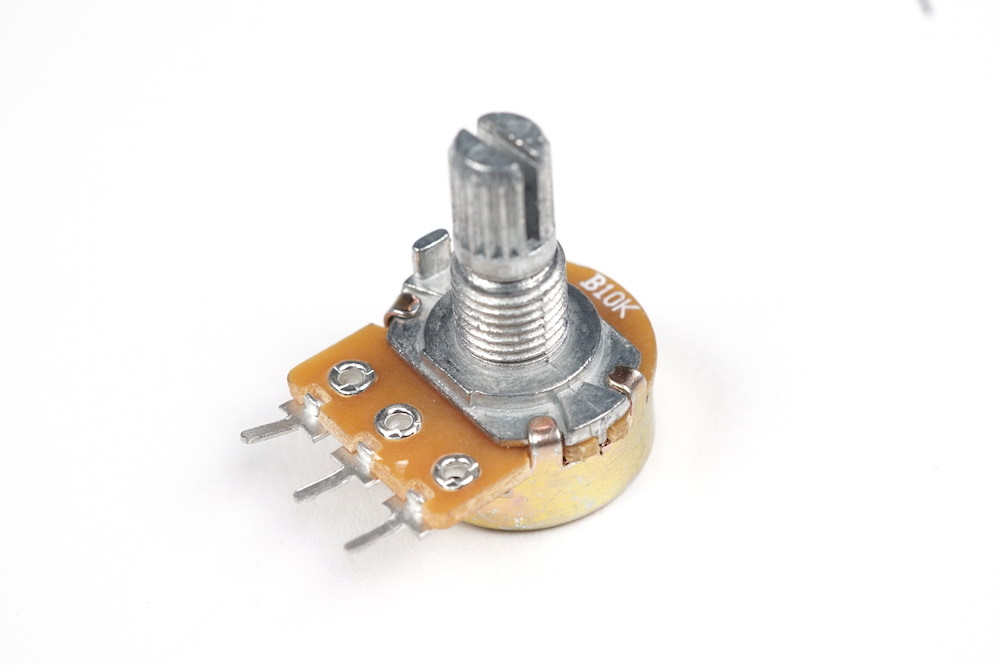Potentiometer
Category:
Subcategory:
Signal Type:
Continuous
IDeATe Component Number:
0390
A rotating variable resistor (knob)What it is

The potentiometer is variable resistor that is adjusted with rotation (a knob).

When to use it

When you need an adjustable knob with a finite range or when you need to measure rotation within a finite range. The potentiometer has a finite range because it may turn all the way to the left and stop and turn all the way to the right and stop.

How it works

A potentiometer is a variable resistor. The potentiometer works by sliding a wiper around a resistive track. The wiper is connected to the center pin, and the outer pins are connected to either end of the track. The closer the wiper is to either end of the track, the smaller the resistance is from the wiper to that end of the track. So, turning the potentiometer all the way to the left would mean there is no resistance between the wiper and the left pin but a lot of resistance between the wiper and the right pin. Moving the potentiometer to the center would cause there to be equal resistances between the wiper and the two pins.

How to use it

The rotation of the potentiometer can be measured with an analog pin on an Arduino. If the left pin is connected to ground and the right pin is connected to 5V, then the middle pin (the wiper) will have a voltage that ranges from 0V to 5V as the potentiometer turns from left to right.

Getting started
``````/*
* This reads a potentiometer on analog pin POTENTIOMETER_PIN
* and sends the data back to the computer via serial.
*
* Created 2021-04-02 by Perry Naseck
*/

// Set which analog pin on the Arduino that the middle pin of
// the potentiometer is connected to
const int POTENTIOMETER_PIN = A0;

// A place to store the data when received
int potentiometerVal = 0;

void setup() {
// Setup serial port to send the data back to the computer
Serial.begin(9600);

// Setup the potentiometer pin as an input
pinMode(POTENTIOMETER_PIN, INPUT);
}

void loop() {
// Get the current potentiometer state (saves a value
// from 0 to 1023)